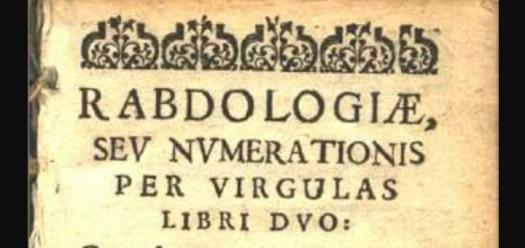# What Do You Know About Rabdology?

Approved & Edited by ProProfs Editorial Team
At ProProfs Quizzes, our dedicated in-house team of experts takes pride in their work. With a sharp eye for detail, they meticulously review each quiz. This ensures that every quiz, taken by over 100 million users, meets our standards of accuracy, clarity, and engagement.
A
Community Contributor
Quizzes Created: 145 | Total Attempts: 37,893
Questions: 10 | Attempts: 115SettingsWritten and published as far back as the 17th century, Rabdology (or its Latin Rabdologiæ) is a treatise by famous Scottish astronomer, mathematician, and physicist named Marvellous Merchiston.
The treatise covers three separate non-logarithm devices to help with arithmetic calculations.
The devices are used to minimize division and multiplication of natural numbers to fundamental subtraction and addition operations.

• 1.

### When was Rabdology invented?

• A.

1916

• B.

1917

• C.

1918

• D.

1919

B. 1917
Explanation
Rabdology was invented in 1917.

Rate this question:

• 2.

### What is Rabdology?

• A.

The method or art of performing arithmetical operations by means of Napier's bones

• B.

The method or art of performing geometrical operations by means of Napier's bones

• C.

The method or art of performing intrinsic operations by means of Napier's bones

• D.

The method or art of performing extrinsic operations by means of Napier's bones

A. The method or art of performing arithmetical operations by means of Napier's bones
Explanation
Rabdology is the method or art of performing arithmetical operations by means of Napier's bones. Napier's bones are a set of numbered rods or bones that can be used to perform multiplication, division, and square root calculations. This method was developed by the Scottish mathematician John Napier in the 17th century as a tool to simplify complex calculations.

Rate this question:

• 3.

### Who invented Rabdology?

• A.

John Napier

• B.

Joshua Napier

• C.

Isaac Napier

• D.

Ezekiel Napier

A. John Napier
Explanation
John Napier is credited with inventing Rabdology, which is the practice of using rods or sticks to perform calculations. He developed the concept of logarithms and introduced the use of logarithmic tables, which greatly simplified complex mathematical calculations. Napier's invention of Rabdology revolutionized the field of mathematics and paved the way for advancements in various scientific disciplines.

Rate this question:

• 4.

### In Rabdology, how many devices do we use for calculations?

• A.

1

• B.

2

• C.

3

• D.

4

C. 3
Explanation
In Rabdology, three devices are used for calculations.

Rate this question:

• 5.

### What is the inscription on the first device?

• A.

Four figure table

• B.

Logarithm table

• C.

Multiplication table

• D.

Saturated steam table

C. Multiplication table
• 6.

### The rods on the first device were used for the following calculation except which one?

• A.

• B.

Multiplication

• C.

Division

• D.

Square root

Explanation
The given question asks about the calculation that was not performed using the rods on the first device. The options provided are addition, multiplication, division, and square root. The correct answer is "Addition" because it implies that the rods on the first device were used for all other calculations such as multiplication, division, and square root, except for addition.

Rate this question:

• 7.

### The distinguishing feature of the second device is its ability to do which of these?

• A.

• B.

Divide numbers

• C.

Subtract numbers

• D.

Square numbers

B. Divide numbers
Explanation
The distinguishing feature of the second device is its ability to divide numbers. This means that the device is capable of performing the mathematical operation of division, where one number is divided by another to obtain a quotient. This feature sets it apart from the other options listed, which involve different mathematical operations such as addition, subtraction, and squaring.

Rate this question:

• 8.

### What was used to represent the second device?

• A.

Promptuary

• B.

Punctured lines

• C.

Rings

• D.

Tyres

A. Promptuary
• 9.

### On the third device, which form is the number converted to?

• A.

Logarithm form

• B.

Binary form

• C.

Matrix form

• D.

Trigonometric form

B. Binary form
Explanation
The correct answer is Binary form. Binary form refers to a number system that uses only two digits, 0 and 1, to represent numbers. In this form, numbers are converted into a series of 0s and 1s, which is commonly used in computer systems and digital electronics.

Rate this question:

• 10.

### The third device can be used to perform the following operation except?

• A.

Multiplication

• B.

Division

• C.

Square root

• D.Back to top